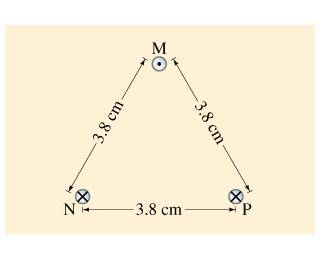# Three wires and currents.

jvdamdshdt

## Homework Statement

In the figure (Figure 1) the top wire is 1.1−mm -diameter copper wire and is suspended in air due to the two magnetic forces from the bottom two wires. The current flow through the two bottom wires is 87A in each.

Find the Current through the Copper wire.## Homework Equations

F/L = I1 I2 U0 /2pi*r

## The Attempt at a Solution

The furthest I've got was to resolve the force on M
2FCos30

F/1 = 2(87^2 * 4pi * 10^-7 / 2*pi*0.038) Cos 30

I don't know where to go with this one.

Last edited:

Enigman
What are you supposed to find?
Anyway:
Calculate the mass per length of the suspended wire, and use the fact that the sum of vertical forces is zero as its in equilibrium.

•1 person
jvdamdshdt
What are you supposed to find?
Anyway:
Calculate the mass per length of the suspended wire, and use the fact that the sum of vertical forces is zero as its in equilibrium.

I am suppose to find the current through the copper wire on top. I tired finding the force and then rearranged the F= BIL formula to find the current.

mass per unit length would be
rho * g * volume?

Last edited:
Enigman
I am suppose to find the current through the copper wire on top. I tired finding the force and then rearranged the F= BIL formula to find the current.
OKay, so current it is, jvdamdshdt!(complicated name...)
There's a current flowing through M and we don't know what that is. What we do know is its suspended in the air, not going up nor down that is force by wires counteract gravity.
mass per unit length would be
rho * g * volume?
Nooooooooo....
You have got the radius and mass per unit length is just mass of wire of 1m length.
You can look up the density of copper and you are ready to go.
:thumbs:

jvdamdshdt
OKay, so current it is, jvdamdshdt!(complicated name...)
There's a current flowing through M and we don't know what that is. What we do know is its suspended in the air, not going up nor down that is force by wires counteract gravity.

Nooooooooo....
You have got the radius and mass per unit length is just mass of wire of 1m length.
You can look up the density of copper and you are ready to go.
:thumbs:

I having trouble understanding. From the Force I calculated 2FCos30, the gravity counteract the force pushing up. Are you saying I need to find the mass and divide that by the length?

Enigman
Yes, and then find the force per unit length by gravity.
(Note: For mass per unit length you can also take length as 1m you will get the same numerical value.
Or more rigorously, you could use M=ρV where V is volume of a cylinder and find M/L ie. mass per unit length)
After that use the fact that sum of forces in vertical direction is zero and hence so too the force per unit length.•1 person
jvdamdshdt
Okay I have M/L = 8940 (rho) * pie * 0.0011^2 = 0.033 kg/m, using this is can find mg/L right?

jvdamdshdt
(F/L) * (2pi *r) / (Unot * I1) = I2

0.33 F/L * (2pi * 0.038m) / (4pi x 10^-7 * 87A) = 727
This is what I did but my answer is 727 A which doesn't seem reasonable.

Enigman
$$0= I_1 I_2 u_o cos\theta/\pi r-mg/L$$
(Try learning ##\LaTeX##)

Last edited:
jvdamdshdt
I couldn't get it but thanks for your help. The answer was 100A and I have no idea where I am plugging the numbers wrong.